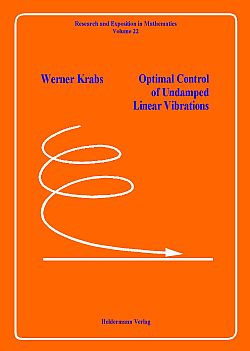R&E 22 Catalogue of allBook Series List of Booksin this Series Previous Book Next Book Research and Exposition in Mathematics -- Volume 22Enlarged Picture W. Krabs Optimal Control of Undamped Linear Vibrations 188 p., soft cover, ISBN 3-88538-222-9, EUR 40.00, 1995 Considered are vibrating systems with finitely many and infinitely many degrees of freedom which are undamped and linear. The first can be described by linear systems of ordinary differential equations of second order without first order terms. The control of the systems is performed by exterior forces on the right hand side of the equations and is called lumped control. In the case of infinitely many degrees of freedom the vibrating systems are described by linear partial differential equations of second order with respect to the time under linear boundary conditions. There are two kinds of control: distributed control where the control is performed by an exterior force on the right hand side of the differential equation and boundary control which is applied to one or more boundary conditions. The main purpose of this book is to investigate the vibrating systems under the aspect of null-controllability. In particular the relationship between time-minimal null-controllability with norm bounded controls and minimum norm null-controls on fixed time intervals is studied because it is the key for a numerical solution. Distributed control of partial differential equations is uniformly treated within the framework of abstract wave equations for Hilbert space valued functions which also comprises the case of lumped control of vibrating systems with finitely many degrees of freedom. The corresponding control problems are approximately solved with the aid of Galerkin's method and by applying duality results for certain minimum norm problems.